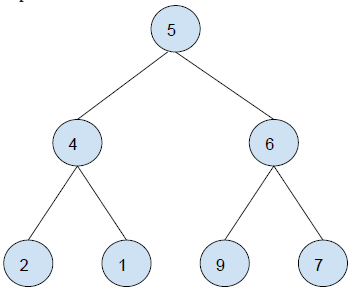# Find sum of all left leaves in a given Binary Tree in C++

In this problem, we are given a binary tree. Our task is to find the sum of all left leaves in a given Binary Tree.

Let's take an example to understand the problem,

Input :Output : 11

Explanation

All leaf nodes of the tree are : 2, 9
Sum = 2 + 9 = 11

## Solution Approach

A simple solution to the problem is traversing the tree from root to leaf. If a node is a left leaf node, add it to sum. When the whole tree is traversed. Print Sum.

## Example

Program to illustrate the working of our solution

#include <iostream>
using namespace std;
struct Node{
int key;
struct Node* left, *right;
};
Node *newNode(char k){
Node *node = new Node;
node->key = k;
node->right = node->left = NULL;
return node;
}
bool isLeafNode(Node *node){
if (node == NULL)
return false;
if (node->left == NULL && node->right == NULL)
return true;
return false;
}
int findLeftLeavesSum(Node *root){
int sum = 0;
if (root != NULL){
if (isLeafNode(root->left))
sum += root->left->key;
else
sum += findLeftLeavesSum(root->left);
sum += findLeftLeavesSum(root->right);
}
return sum;
}
int main(){
struct Node *root = newNode(5);
root->left = newNode(4);
root->right = newNode(6);
root->left->left = newNode(2);
root->left->right = newNode(1);
root->right->left = newNode(9);
root->right->right = newNode(7);
cout<<"The sum of left leaves of the tree is "<<findLeftLeavesSum(root);
return 0;
}

## Output

The sum of left leaves of the tree is 11

Another approach using Iteration

We will perform depth first search traversal on the tree, And then check if the current node is a left leaf. If Yes, add its value to sum, otherwise, leave. At the end, print the sum.

## Example

Program to illustrate the working of our solution

#include<bits/stdc++.h>
using namespace std;
struct Node{
int key;
struct Node* left, *right;
};
Node *newNode(char k){
Node *node = new Node;
node->key = k;
node->right = node->left = NULL;
return node;
}
int findLeftLeavesSum(Node* root){
if(root == NULL)
return 0;
stack<Node*> treeNodes;
treeNodes.push(root);
int sum = 0;
while(treeNodes.size() > 0){
Node* currentNode = treeNodes.top();
treeNodes.pop();
if (currentNode->left != NULL){
treeNodes.push(currentNode->left);
if(currentNode->left->left == NULL &&
currentNode->left->right == NULL){
sum += currentNode->left->key ;
}
}
if (currentNode->right != NULL)
treeNodes.push(currentNode->right);
}
return sum;
}
int main(){
Node *root = newNode(5);
root->left= newNode(4);
root->right = newNode(6);
root->left->left = newNode(2);
root->left->right = newNode(1);
root->right->left = newNode(9);
root->right->right= newNode(7);
cout<<"The sum of left leaves of the tree is "<<findLeftLeavesSum(root);
return 0;
}

## Output

The sum of left leaves of the tree is 11

Approach 3, using BFS

We will perform a breadth first search with a variable to indicate whether the node is left child or not. If it is, add it to the sum, else leave. At the end, print sum.

## Example

Program to illustrate the working of our solution

#include<bits/stdc++.h>
using namespace std;
struct Node{
int key; struct Node* left, *right;
};
Node *newNode(char k){
Node *node = new Node;
node->key = k;
node->right = node->left = NULL;
return node;
}
int findLeftLeavesSum(Node* root) {
if (root == NULL)
return 0;
queue<pair<Node*, bool> > leftTreeNodes;
leftTreeNodes.push({ root, 0 });
int sum = 0;
while (!leftTreeNodes.empty()) {
Node* temp = leftTreeNodes.front().first;
bool is_left_child = leftTreeNodes.front().second;
leftTreeNodes.pop();
if (!temp->left && !temp->right && is_left_child)
sum = sum + temp->key;
if (temp->left) {
leftTreeNodes.push({ temp->left, 1 });
}
if (temp->right) {
leftTreeNodes.push({ temp->right, 0 });
}
}
return sum;
}
int main(){
Node *root = newNode(5);
root->left= newNode(4);
root->right = newNode(6);
root->left->left = newNode(2);
root->left->right = newNode(1);
root->right->left = newNode(9);
root->right->right= newNode(7);
cout<<"The sum of left leaves of the tree is "<<findLeftLeavesSum(root);
return 0;
}

## Output

The sum of left leaves of the tree is 11